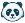# [New Quizzes] Grouped Formula Questions - Shared Set of Defined Variables

The formula based questions are an essential tool for math based courses, but there is a big limiting factor. Formula questions work on a set of defined variables input by the teacher. I would like to be able to ask multiple, related formula-based questions with a shared set of defined variables.

Ex:

A ball of mass 'm' kg is on Earth, what is its mass?

The ball of mass 'm' kg is on Moon, what is its mass?

The ball of mass 'm' kg is floating in space, what is its mass?

A ball of mass 'm' kg is on Earth, what is its weight?

The ball of mass 'm' kg is on Moon, what is its weight?

The ball of mass 'm' kg is floating in space, what is its weight?

I would like to be able to ask these six questions about the SAME ball of mass 'm' and use the formula to generate multiple iterations. As is, to do this using formula questions, the questions need to be input as 6 separate formula-based questions. The result is that the ball will have a different mass for all six questions and it makes follow up questions more challenging.

I have thought of three possible solutions to solve this depending on what is technically possible:

1. A formula question group
• Under the question group feature, define a set of variables that applies to all the questions in the group.
2. Multiple formula questions
• Under a single formula question, once the variables are defined, allow the input of multiple formulas for different questions
3. The new stimulus-response feature in new quizzes
• Allow the stimulus column to be input with a formula-based question, and then carry the defined variables over into the response questions

This feature would be a HUGE help for our math/science teachers and students!Community MemberCommunity Member

A quiz question type that allows for students to input data and have their future calculations checked based on that input.

As an example:

- Having students input the length and width of a shape in Questions 1 and 2

- Calculating area based on their answers for Question 3

That way a teacher can pass out different sizes and the canvas quiz automatically checks it against their measurements. Thus checking the process of solving a question and not seeing if they can just get specific numbers.Community Novice

Yes, this would be great! I would like to be able to establish one or more variables in a stimulus that can be used in any formula questions attached to that stimulus. This would greatly increase my ability to assess depth of knowledge. It would be a huge improvement. For now I am forced to use other tools that offer that feature.Community Member

This would be a wonderful addition to a math, science or statistics class (among other classes).Community Team
Status changed to: NewCommunity Team
Status changed to: NewCommunity Team
Status changed to: Added to Theme